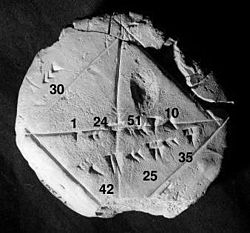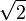# Numerical analysis facts for kids

Kids Encyclopedia FactsBabylonian clay tablet YBC 7289 (c. 1800–1600 BC) with annotations. The approximation of the square root of 2 is four sexagesimal figures, which is about six decimal figures. 1 + 24/60 + 51/602 + 10/603 = 1.41421296...

Numerical analysis studies different algorithms to get approximations for problems of mathematical analysis. Approximations are used for the following reasons:

1. There are no known ways to solve a problem using calculus. Examples for such problems are the Navier–Stokes equations or the Three-body problem
2. There is a way to solve a problem using calculus. Getting an exact solution is impractical though, because it requires a long time, or many resources. An example for this is calculating Power series.

One of the earliest known uses of numerical analysis is a Babylonian clay tablet, which approximates the square root of 2. In a unit square, the diagonal has this length. Being able to compute the sides of a triangle is extremely important, for instance, in carpentry and construction.

Numerical analysis continues this long tradition of practical mathematical calculations. Much like the Babylonian approximation of$\sqrt{2}$, modern numerical analysis does not seek exact answers, because exact answers are often impossible to obtain in practice. Instead, much of numerical analysis is concerned with obtaining approximate solutions while maintaining reasonable bounds on errors.

Numerical analysis naturally finds applications in all fields of engineering and the physical sciences, but in the 21st century, the life sciences and even the arts have adopted elements of scientific computations. Ordinary differential equations appear in star movement; optimization occurs in portfolio management; numerical linear algebra is important for data analysis; stochastic differential equations and Markov chains are essential in simulating living cells for medicine and biology.

Computers greatly helped this task. Before there were computers, numerical methods often depended on hand interpolation in large printed tables. Since the mid 20th century, computers calculate the required functions instead. These same interpolation formulas nevertheless continue to be used as part of the software algorithms for solving differential equations.Numerical analysis Facts for Kids. Kiddle Encyclopedia.